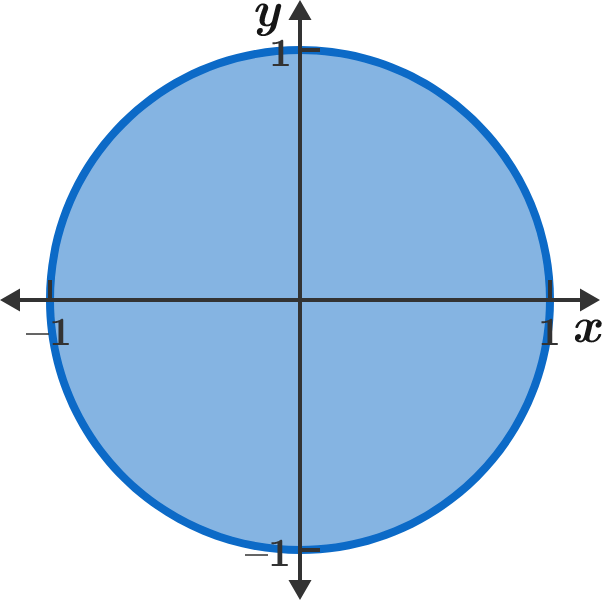# An Area Worth Exploring

Geometry Level 2It's common knowledge that the graph of $x^2 + y^2 \leq 1$ is a unit disk, and the area of the region is $\pi.$

However, it's not common for someone to know the shape of the graph $\big\lfloor x^2 \big\rfloor + \big\lfloor y^2 \big\rfloor = 1,$ where $\lfloor \cdot \rfloor$ is the floor function. To three decimal places, what is the area of the region on the coordinate plane that satisfies the equation $\big\lfloor x^2 \big\rfloor + \big\lfloor y^2 \big\rfloor = 1?$


Note: Try to draw a hand sketch of this curve and provide analysis instead of using any software!

×